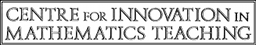Multiple Choice Questions
Unit G4
Functions
For each of these questions, choose the option that is TRUE.
1

If f(x) = 3x2 + 4x − 5, then f(−2) =

• (A)
−49
• (B)
−1
• (C)
9
• (D)
23
2

1   →   3

2   →   5

3   →   7

4   →   9

Which of the following could describe the mapping above?

• (A)
f : xx + 3
• (B)
f : x → 2x + 1
• (C)
f : xx2 + 1
• (D)
f : x → 2x2 − 3
3

If f : xx2 + 1, then f(−3) is

• (A)
10
• (B)
7
• (C)
−5
• (D)
−8
4

f(4) = −2, so f−1(−2) =

• (A)
−4
• (B)
• (C)
• (D)
4
5

If f(x) = x + 1 and g(x) = x2 , then gf(2) =

• (A)
3
• (B)
4
• (C)
5
• (D)
9
6

f(x) = 2x − 1, so f−1(3) =

• (A)
1
• (B)
2
• (C)
3
• (D)
4
7

f(x) = , ff−1(1) =

• (A)
2
• (B)
1
• (C)
• (D)
0
Total: - of marks